﻿ MotionDesigner Reference & User Interface > Motion Laws > Y-Inverse-Sinusoid [Special]

# Motion-Law: Y–Inverse-Sinusoid

Navigation:  MotionDesigner Reference & User Interface > Motion Laws >

# Motion-Law: Y–Inverse-Sinusoid

### Y–Inverse-Sinusoid

Note: This Motion-Law is usually applied to a Rotating Crank.

You can use this segment one time per motion.

We recommend that you use the Constant-Velocity Inverse Crank, as it can be used many times per motion.

 This segment is best explained by considering the X and Y motion components of a Point that is at the end of a rotating-Part or Crank. When a Crank rotates with Constant angular velocity, the motion of a Point at the end of the Crank that is projected onto a Line is Simple-Harmonic-Motion ( a Sine function ) When you apply the Y–Inverse Sinusoid segment to a Crank, the motion of a Point at the end of the Crank that is projected onto a Line can be made to move with a constant linear velocity for a phase of the Crank's rotation.Y-Inverse-Sinusoid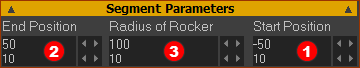Example Segment Parameters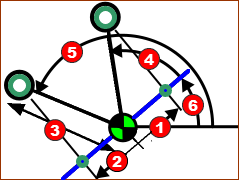Schematic of Crank made to give a constant linear velocity ... Schematic of Crank made to give a constant linear velocity from angle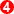, defined by Start-Position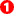, to angle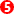, defined by the End-Position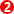. MechDesigner calculates anglesand. Angle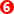is the Base-Value in the Motion-Dimension FB. Use Segment-Parameters to enter the Start-Position, End-Position, and  is the Radius of the Rocker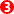. Segment Parameters. Radius of Rocker* (Example is 100) (* When the Rocker rotates for a full rotation, we can also use the term Crank). The Radius of the Rocker specifies the minimum and maximum range of positions when projected onto a Line. Start-Position (Example SP=-50) (Note: Do not confuse this with the Start-Position in the Segment-Editor) Position of the Point that is projected onto a reference Line when it start to move at Constant-Velocity. MechDesigner calculates the Angle of the Crank to give this distance, relative to the Reference Line of the Crank. End-Position (Example EP = -50)  (Note: Do not confuse this with the End-Position in the Segment-Editor) At the end of the Constant-Velocity, End-Position specifies the distance from the end of the Crank to its Center, when projected onto the reference Line* of the Crank. MechDesigner calculates the Angle of the Crank to give this distance, relative to the Reference Line of the Crank.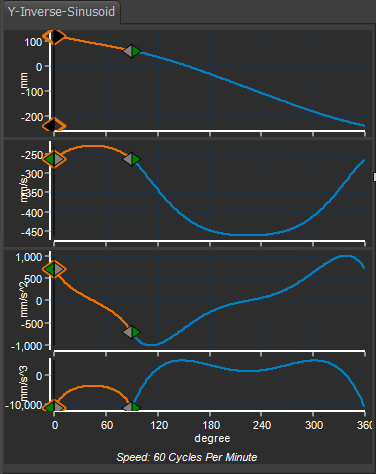Y-Inverse Sinusoid Example: with two segments. Crank rotates in a Counter-Clockwise direction Constant-Velocity Starts at 50mm to Left of Crank-Center (angle 120º) Constant-Velocity Ends at 50 to Right of Crank-Center (angle 60º) Segment 1: •X-axis Start = 0 ; End = 90º The Y–Inverse Sinusoid Segment-Parameters are: •Radius of Rocker = 100mm, Start Position = -50, End Position = 50mm. With these Segment-Parameters: •The Y–axis is 120º†, when the Master Machine Angle (MMA) is 0º . •The Y–axis is 60º, when the Master Machine Angle is 90º . Segment 2: •X-axis Start = 90º, End = 360º (0º) A Flexible-Polynomial •The Y–axis is 60º, when the Master Machine Angle is 90º (= end of Segment 1) •The Y–axis –240º when the Master Machine Angle is 360º . Thus the Crank makes a full rotation from +120 to -240 in a counter-clockwise direction.